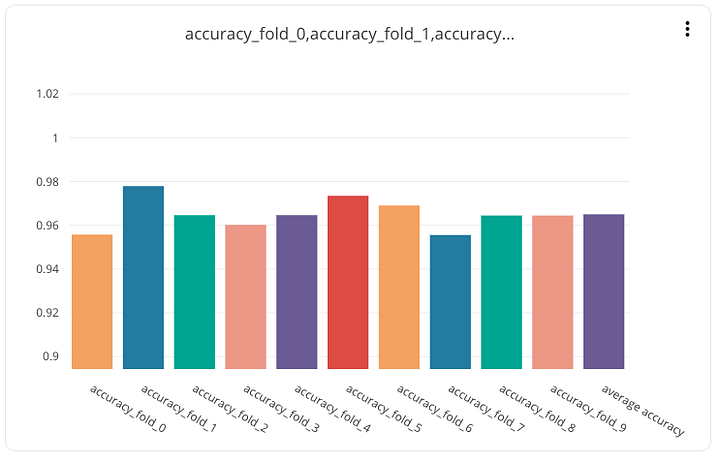# Building Reliable Machine Learning Models with Cross-validation

Cross-validation is frequently used to train, measure and finally select a machine learning model for a given dataset because it helps assess how the results of a model will generalize to an independent data set in practice.comments

By Gideon Mendels, Co-Founder and CEO @ Comet.ml

Cross-validation is a technique used to measure and evaluate machine learning models performance. During training we create a number of partitions of the training set and train/test on different subsets of those partitions.

Cross-validation is frequently used to train, measure and finally select a machine learning model for a given dataset because it helps assess how the results of a model will generalize to an independent data set in practice. Most importantly, cross-validation has been shown to produce models with lower bias than other methods.
This tutorial will focus on one variant of cross-validation named k-fold cross-validation.

In this tutorial we’ll cover the following:

1. Overview of K-Fold Cross-Validation
2. Example using Scikit-Learn and Comet.ml

### K-Fold Cross-Validation

Cross-validation is a resampling technique used to evaluate machine learning models on a limited data set.

The most common use of cross-validation is the k-fold cross-validation method. Our training set is split into K partitions, the model is trained on K-1 partitions and the test error is predicted and computed on the Kth partition. This is repeated for each unique group and the test errors are averaged across.

The same procedure is described by the following steps:

1. Split the training set into K (K=10 is a common choice) partitions

For each partition:

1. Set the partition is the test set
2. Train a model on the rest of the partitions
3. Measure performance on the test set
4. Retain the performance metric
5. Explore model performance over different folds

Cross-validation is commonly used since it’s easy to interpret and since it generally results in a less biased or less optimistic estimates of the model performance than other methods, such as a simple train/test split. One of the biggest downsides in using cross-validation is the increased training time as we are essentially training K times instead of 1.

### Cross-validation example using scikit-learn

Scikit-learn is a popular machine learning library that also provides many tools for data sampling, model evaluation and training. We’ll use the `Kfold`class to generate our folds. Here’s a basic overview:

```from sklearn.model_selection import KFold
X = [...] # My training dataset inputs/features
y = [...] # My training dataset targets

kf = KFold(n_splits=2)
kf.get_n_splits(X)

for train_index, test_index in kf.split(X):
X_train, X_test = X[train_index], X[test_index]
y_train, y_test = y[train_index], y[test_index]
model = train_model(X_train,y_train)
score = eval_model(X_test,y_test)```

### Now let’s train an end-to-end example using scikit-learn and Comet.ml.

This example trains a text classifier on the news groups dataset (you can find it here). Given a piece of text (string), the model classifies it to one of the following classes: “atheism”,”christian”,”computer graphics”, “medicine”.

On every fold we report the accuracy to Comet.ml and finally we report the average accuracy of all folds. After the experiment finishes, we can visit Comet.ml and examine our model:The following chart was automatically generated by Comet.ml. The right most bar (in purple) represents the average accuracy across folds. As you can see some folds preform significantly better than the average and shows how important k-fold cross validation is.

You might have noticed that we didn’t compute the test accuracy. The test set should not be used in any way until you’re completely finished with all experimentation. If we change hyperparameters or model types based on the test accuracy we’re essentially over-fitting our hyperparameters to the test distribution.

Still curious about cross-validation? Here are some other great resources:

Thanks to Cecelia Shao.

Bio: Gideon Mendels is Co-Founder and CEO @ Comet.ml.

Comet.ml is doing for ML what Github did for code. Our lightweight SDK enables data science teams to automatically track their datasets, code changes, experimentation history. This way, data scientists can easily reproduce their models and collaborate on model iteration amongst their team!

Original. Reposted with permission.

Related: